This paper introduces the basics of designing a digital radio receiver. With many new advances in data converter and radio technology, complex receiver design has been greatly simplified. This paper attempts to explain how to calculate sensitivity and selectivity of such a receiver. It is not by any means an exhaustive exposition, but is instead a primer on many of the techniques and calculations involved in such designs.

Many advances in radio design and architecture are now allowing for rapid changes in the field of radio design. These changes allow reduction of size, cost, complexity and improve manufacturing by using digital components to replace unreliable and in-accurate analog components. For this to happen, many advances in semiconductor design and fabrication were required and have come to fruition over the last few years. Some of these advances include better integrated mixers, LNA, improved SAW filters, lower cost high performance ADCs and programmable digital tuners and filters. This article summarizes the design issues with and the interfacing of these devices into complete radio systems.

Traditionally, a radio has been considered to be the ‘box’ that connects to the antenna and everything behind that, however, many system designs are segmented into two separate subsystems. The radio and the digital processor. With this segmentation, the purpose of the radio is to down convert and filter the desired signal and then digitize the information. Likewise, the purpose of the digital processor is to take the digitized data and extract out the desired information.

An important point to understand is that a digital receiver is not the same thing as digital radio(modulation). In fact, a digital receiver will do an excellent job at receiving any analog signal such as AM or FM. Digital receivers can be used to receive any type of modulation including any analog or digital modulation standards. Furthermore, since the core of the digital processor is a digital signal processor (DSP), this allows many aspects of the entire radio receiver itself be controlled through software. As such, these DSPs can be reprogrammed with upgrades or new features based on customer segmentation, all using the same hardware. However, this is a complete discussion in itself and not the focus of this article.

1. Available Noise Power
4. Conversion Gain and Sensitivity
5. ADC Spurious Signals and Dither
6. Third Order Intercept Point
8. Phase Noise
9. IP3 in the RF section

### Single-Carrier vs. Multi-Carrier

Before a detailed discussion of designing a digital radio receiver are discussed, some of the technical benefits need to be discussed. These include Oversampling, Processing Gain, Undersampling, Frequency planning/Spur placement. Many of these provide technical advantages not otherwise achievable with a traditional radio receiver design.

### Over Sampling and Process Gain

The Nyquist criterion compactly determines the sample rate required for any given signal.  Many times, the Nyquist rate is quoted as the sample rate that is twice that of the highest frequency component. This implies that for an IF sampling application at 70 MHz, a sample rate of 140 MSPS would be required.  If our signal only occupies 5 MHz around 70 MHz, then sampling at 140 MSPS is all but wasted.  Instead, Nyquist requires that the signal be sampled twice the bandwidth of the signal. Therefore, if our signal bandwidth is 5 MHz, then sampling at 10 MHz is adequate.  Anything beyond this is called Over Sampling. Oversampling is a very important function because it allows for an effective gain of received SNR  in the digital domain.

In contrast to over sampling is the act of under sampling. Under sampling is the act of sampling at a frequency much less than the half of the actual signal frequency (See the section below on undersampling). Therefore, it is possible to be oversampling and undersampling simultaneously since one is defined with respect to bandwidth and the other at the frequency on interest.

In any digitization process, the faster that the signal is sampled, the lower the noise floor because noise is spread out over more frequencies. The total integrated noise remains constant but is now spread out over more frequencies which has benefits if the ADC is followed by a digital filter.  The noise floor follows the equation: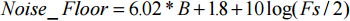This equation represents the level of the quantization noise within the converter and shows the relationship between noise and the sample rate FS.  Therefore each time the sample rate is doubled, the effective noise floor improves by 3 dB!

Digital filtering has the effect of removing all unwanted noise and spurious signals, leaving only the desired signal as shown in the figures below.

SNR of the ADC may be greatly improved as shown in the diagram above. In fact, the SNR can be improved by using the following equation: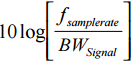As shown, the greater the ratio between sample rate and signal bandwidth, the higher the process gain. In fact, gains as high as 30 dB are achievable.

### Undersampling and Frequency Translation

As stated earlier, under sampling is the act of sampling at a frequency much less than the half of the actual signal frequency. For example, a 70 MHz signal sampled at 13 MSPS is an example of undersampling.

Under sampling is important because it can serve a function very similar to mixing. When a signal is under sampled, the frequencies are aliased into baseband or the first Nyquist zone as if they were in the baseband originally. For example, our 70 MHz signal above when sampled at 13 MSPS would appear at 5 MHz.  This can mathematically be described by:This equation provides the resulting frequency in the first and second Nyquist zone.  Since the ADC aliases all information to the first Nyquist zone, results generated by this equation must be checked to see if they are above fSampleRate/2 .  If they are, then the frequency must be folded back into the first Nyquist zone by subtracting the result from fSampleRate.

The table below shows how signals can be aliased into baseband and their spectral orientation. Although the process of sampling (aliasing) is different than mixing (multiplication), the results are quite similar, but periodic about the sample rate. Another phenomenon is that of spectral reversal. As in mixers, certain products become reversed in the sampling process such as upper and lower sideband reversal.  The table below also shows which cases cause spectral reversal.

 Input Signal Frequency Range Frequency Shift Spectral Sense 1st Nyquist Zone DC - FS/2 Input Normal 2nd Nyquist Zone FS/2 - FS FS-Input Reversed 3rd Nyquist Zone FS - 3FS/2 Input - FS Normal 4th Nyquist Zone 3FS/2 - 2FS 2FS - Input Reversed 5th Nyquist Zone 2FS - 5FS/2 Input - 2FS Normal

Frequency Planning and Spur Placement

One of the biggest challenges when designing a radio architecture is that of IF frequency placement.  Compounding this problem is that drive amplifiers and ADCs tend to generate unwanted harmonics that show up in the digital spectrum of the data conversion, appearing as false signals. Whether the application is wideband or not, careful selection of sample rates and IF frequencies can place these spurs at locations that will render them harmless when used with a digital tuners/filters, like the AD6620,  that can select the signal of interest and reject all others. All of this is good, because by carefully selecting input frequency range and sample rate, the drive amplifier and ADC harmonics can actually be placed out-of-band. Oversampling only simplifies matters by providing more spectrum for the harmonics to fall harmlessly within.

For example, if the second and third harmonics are determined to be especially high, by carefully selecting where the analog signal falls with respect to the sample rate, these second and third harmonics can be placed out-of-band.  For the case of an encode rate equal to 40.96 MSPS and a signal bandwidth of 5.12 MHz, placing the IF between 5.12 and 10.24 MHz places the second and third harmonics out of band as shown in the table below.  Although this example is a very simple, it can be tailored to suit many differed applications.

As can be seen, the second and third harmonics fall away from the band of interest and cause no interference to the fundamental components. It should be noted that the seconds and thirds do overlap with one another and the thirds alias around FS/2.  In tabular for this looks as shown below.

 Encode Rate: 40.96 MSPS Fundamental 5.12 - 10.24 MHz Second Harmonic: 10.24 - 20.48 MHz Third Harmonic: 15.36 - 10.24 MHz

Another example of frequency planning can be found in undersampling. If the analog input signal range is from DC to FS/2 then the amplifier and filter combination must perform to the specification required. However, if the signal is placed in the third Nyquist zone (FS to 3FS/2), the amplifier is no longer required to meet the harmonic performance required by the system specifications since all harmonics would fall outside the passband filter.  For example, the passband filter would range from FS to 3FS/2. The second harmonic would span from 2FS to 3FS, well outside the passband filters range. The burden then has been passed off to the filter design provided that the ADC meets the basic specifications at the frequency of interest.  In many applications, this is a worthwhile tradeoff since many complex filters can easily be realized using SAW and LCR techniques alike at these relatively high IF frequencies. Although harmonic performance of the drive amplifier is relaxed by this technique, intermodulation performance cannot be sacrificed.

Using this technique to cause harmonics to fall outside the Nyquist zone of interest allows them to be easily filtered as shown above. However, if the ADC still generates harmonics of their own, the technique previously discussed can be used to carefully select sample rate and analog frequency so that harmonics fall into unused sections of bandwidth and digitally filtered.

For the discussion that follows, the generic receiver design is shown above. Considered in this discussion begins with the antenna and ends with the digital tuner/filter at the end. Beyond this point is the digital processor which is outside the scope of this discussion.

Analysis starts with several assumptions. First, it is assumed that the receiver is noise limited.  That is that no spurs exist inband that would otherwise limit performance.  It is reasonable to assume that LO and IF choices can be made such that this is true. Additionally, it will be shown later that spurs generated with-in the ADC are generally not a problem as they can often be eliminated with the application of dither or through judicious use of oversampling and signal placement. In some instances, these may not be realistic assumption but they do provide a starting point with which performance limits can be bench marked.

The second assumption is that the bandwidth of the receiver front end is our Nyquist bandwidth. Although our actual allocated  bandwidth may only be 5 MHz, using the Nyquist bandwidth will simplify computations along the way. Therefore, a sample rate of 65 MSPS would give a Nyquist bandwidth of 32.5 MHz.

### Available Noise Power

To start the analysis, the noise at the antenna port must be considered. Since a properly matched antenna is apparently resistive, the following equation can be used to determine the noise voltage across the matched input terminals.Available power from the source, in this case, the antenna is thus:Which simplifies when the previous equation is substituted in to:Thus in reality, the available noise power from the source in this case is independent of impedance for non-zero and finite resistance values.

This is important because this is the reference point with which our receiver will be compared. It is often stated when dealing with noise figure of a stage, that it exhibits ‘x’ dB above ‘kT’ noise. This is the source of this expression.

With each progressive stage through the receiver, this noise is degraded by the noise figure of the stage as discussed below. Finally, when the channel is tuned and filtered, much of the noise is removed, leaving only that which lies within the channel of interest.

Noise figure is a figure of merit used to describe how much noise is added to a signal in the receive chain of a radio. Usually, it is specified in dB although in the computation of noise figure, the numerical ratio (non-log) is used. The nonlog is called Noise factor and is usually denoted as F , where it is defined as shown below.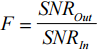Once a noise figure is assigned to each of the stages in a radio, they can be used to determine their cascaded performances. The total noise factor referenced to the input port can be computed as follows.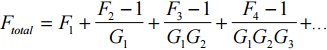The F ’s above are the noise factors for each of the serial stages while the G’s are the gains of the stages. Neither the noise factor or the gains are in log form at this point. When this equation is applied, this reflects all component noise to the antenna port. Thus, the available noise from the previous section can be degraded directly using the noise figure.For example, if the available noise is -100 dBm, the computed noise figure is 10 dB, and conversion gain is 20 dB, then the total equivalent noise at the output is -70 dBm.

There are several points to consider when applying these equations. First, passive components assume that the noise figure is equal to their loss. Second, passive components in series can be summed before the equation is applied. For example if two low pass filters are in series, each with an insertion loss of 3 dB, they may be combined and the loss of the single element assumed to be 6 dB. Finally, mixers often do not have a noise figure assigned to them by the manufacturer. If not specified, the insertion loss may be used, however, if a noise figure is supplied with the device, it should be used.

Although a noise figure could be assigned to the ADC, it is often easier to work the ADC in a different manner. ADC’s are voltage devices, whereas noise figure is really a noise power issue. Therefore, it is often easier to work the analog sections to the ADC in terms of noise figure and then convert to voltage at the ADC. Then work the ADC’s noise into an input referenced voltage. Then, the noise from the analog and ADC can be summed at the ADC input to find the total effective noise.

For this application, an ADC such as the AD9042 or AD6640 12 bit analog to digital converter has been selected. These products can sample up to 65 MSPS, a rate suitable for entire band AMPS digitization and capable of GSM 5x reference clock rate. This is more than adequate for AMPS, GSM and CDMA applications. From the datasheet, the typical SNR is given to be 68dB. Therefore, the next step is to figure the noise degradation within the receiver due to ADC noises. Again, the simplest method is to convert both the SNR and receiver noise into rms. volts and then sum them for the total rms. noise. If an ADC has a 2 volt peak to peak input range: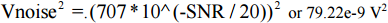This voltage represents all noises within the ADC, thermal and quantization. The full scale range of the ADC is .707 volts rms.

With the ADC equivalent input noise computed, the next computation is the noise generated from the receiver itself. Since we are assuming that the receiver bandwidth is the Nyquist bandwidth, a sample rate of 65 MSPS produces a bandwidth of 32.5 MHz. From the available noise power equations, noise power from the analog front end is 134.55E15 watts or -98.7 dBm. This is the noise present at the antenna and must be gained up by the conversion gain and degraded by the noise figure. If conversion gain is 25 dB and the noise figure is 5 dB, then the noise presented to the ADC input network is:Into 50 ohms (134.9e-12 Watts). Since the ADC has an input impedance of about 1000 ohms, we must either match the standard 50 ohm IF impedance to this or pad the ADC impedance down. A reasonable compromise is to pad the range down to 200 ohms with a parallel resistor and then use a 1:4 transformer to match the rest. The transformer also serves to convert the un-balanced input to the balanced signal required for the ADC as well as provide some voltage gain. Since there is a 1:4 impedance step up, there is also a voltage gain of 2 in the process.From this equation, our voltage squared into 50 ohms is 6.745e-9 or into 200 ohms, 26.98e-9.

Now that we know the noise from the ADC and the RF front end, the total noise in the system can be computed by the square root of the sum of the squares. The total voltage is thus 325.9 uV. This is now the total noise present in the ADC due to both receiver noise and ADC noise, including quantization noise.

### Conversion Gain and Sensitivity

How does this noise voltage contribute to the overall performance of the ADC? Assume that only one RF signal is present in the receiver bandwidth. The signal to noise ratio would then be:Since this is an oversampling application and the actual signal bandwidth is much less than the sample rate, noise will be greatly reduced once digitally filtered. Since the front end bandwidth is the same as our ADC bandwidth, both ADC noise and RF/IF noise will improve at the same rate. Since many communications standards support narrow channel bandwidths, we’ll assume a 30 kHz channel. Therefore, we gain 33.4 dB from process gain. Therefore, our original SNR of 66.7 dB is now 100.1 dB.  Remember, that SNR increased because excess noise was filtered, that is the source of process gain.

If this is a multi-carrier radio, the ADC dynamic range must be shared with other RF carriers. For example, if there are eight carriers of equal power, each signal should be no larger than 1/8th the total range if peak to peak signals are considered. However, since normally the signals are not in phase with one another in a receiver (because remotes are not phase locked), the signals will rarely if ever align. Therefore, much less than the required 18 dB are required. Since in reality, only no more than 2 signals will align at any one time and because they are modulated signals, only 3 dB will be reserved for the purpose of head room. In the event that signals do align and cause the converter to clip, it will occur for only a small fraction of a second before the overdrive condition is cleared.  In the case of a single carrier radio, no head room is required.

Depending on the modulation scheme, a minimum C/N is required for adequate demodulation. If the scheme is digital, then the bit error rate (BER) must be considered as shown below. Assuming a minimum C/N of 10 dB is required, our input signal level can not be so small that the remaining SNR is less than 10 dB. Thus our signal level may fall 90.1 dB from its present level. Since the ADC has a full-scale range of +4 dBm (200 ohms), the signal level at the ADC input is then –86.1 dBm.  If there were 25 dB of gain in the RF/IF path, then receiver sensitivity at the antenna would be –86.1 minus 25 dB or –111.1 dBm.  If more sensitivity is required, then more gain can be run in the RF/IF stages. However, noise figure is not independent of gain and an increase in the gain may also have an adverse effect on noise performance from additional gain stages.

### ADC Spurious Signals & Dither

A noise limited example does not adequately demonstrate the true limitations in a receiver.  Other limitations such as SFDR are more restrictive than SNR and noise.  Assume that the analog-to-digital converter has an SFDR specification of -80 dBFS or -76 dBm (Full-scale = +4dBm).  Also assume that a tolerable Carrier to Interferer, C/I (different from C/N) ratio is 18 dB.  This means that the minimum signal level is -62 dBFS (-80 plus 18) or -58 dBm.  At the antenna, this is -83 dBm. Therefore, as can be seen, SFDR (single or multi-tone) would limit receiver performance long before the actual noise limitation is reached.

However, a technique known as dither can greatly improve SFDR. As shown in Analog Devices Application note AN410, the addition of out of band noise can improve SFDR well into the noise floor.  Although the amount of dither is converter specific, the technique applies to all ADCs as long as static DNL is the performance limitation and not AC problems such as slew rate. In the AD9042 documented in the application note, the amount of noise added is only -32.5 dBm or 21 codes rms. As shown below, the plots both before and after dither provide insight into the potential for improvement. In simple terms, dither works by taking the coherent spurious signals generated within the ADC and randomizes them. Since the energy of the spurs must be conserved, dither simply causes them to appear as additional noise in the floor of the converter.  This can be observed in the before and after plots of dither as a slight increase in the average noise floor of the converter. Thus, the trade off made through the use of out of band dither is that literally all internally generated spurious signals can be removed, however, there is a slight hit in the overall SNR of the converter which in practical terms amounts to less than 1 dB of sensitivity loss compared to the noise limited example and much better than the SFDR limited example shown earlier.

Two important points about dither before the topic is closed. First, in a multi-carrier receiver, none of the channels can be expected to be correlated.  If this is true, then often the multiple signals will serve as self dither for the receiver channel. While this is true some of the time, there will be times when additional dither will need to be added to fill when signal strengths are weak.

Second, the noise contributed from the analog front end alone is insufficient to dither the ADC.  From the example above, 32.5 dBm of dither was added to yield an optimum improvement in SFDR. In comparison, the analog front end only provide –68 dBm of noise power, far from what is needed to provide optimum performance.

### Third Order Intercept Point

Besides converter SFDR, the RF section contributes to the spurious performance of the receiver.  These spurs are unaffected by techniques such as dither and must be addressed to prevent disruption of receiver performance. Third order intercept is an important measure as the signal levels within the receive chain increase  through the receiver design.

In order to understand what level of performance is required of wideband RF components, we will review the GSM specification, perhaps the most demanding of receiver applications.

A GSM receiver must be able to recover a signal with a power level between -13 dBm and -104 dBm. Assume also that the full-scale of the ADC is 0 dBm and that losses through the receiver filters and mixers is 12 dB. Also, since multiple signals are to be processed simultaneously, an AGC should not be employed.  This would reduce RF sensitivity and cause the weaker signal to be dropped.  Working with this information, RF/IF gain is calculated to be 25 dB (0=-13-6-6+x).

The 25 dB gain require is distributed as shown. Although a complete system would have additional components, this will serve this discussion. From this, with a full-scale GSM signal at -13 dBm, ADC input will be 0 dBm.  However, with a minimal GSM signal of -104 dBm, the signal at the ADC would be -91 dBm. From this point, the discussion above can be used to determine the suitability of the ADC in terms of noise performance and spurious performance.

Now with these signals and the system gains required, the amplifier and mixer specifications can now be examined when driven by the full-scale signal of -13 dBm. Solving for the 3rd order products in terms of signal full-scale: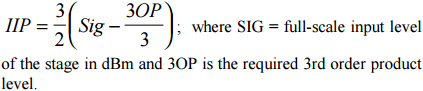Assuming that overall spurious performance must be greater than 100 dB, solving this equation for the front end amplifier shows that a third order input amplifier with a IIP>+37 dBm. At the mixer, the signal level as been gained by 10 dB, and the new signal level is -3 dBm. However, since mixers are specified at their output, this level is reduced by at least 6 dB to –9 dBm.  Therefore for the mixer, a OIP>+41 dBm.  Since mixers are specified at their output. At the final gain stage, the signal will be attenuated to -9 dBm (Same as the mixer output).  For the IF amplifier, the IIP>+41 dBm. If these specifications are met, then the performance should be equal to

One dynamic specification that is vital to good radio performance is ADC clock jitter. Although low jitter is important for excellent base band performance, its effect is magnified when sampling higher frequency signals (higher slew rate) such as is found in undersampling applications. The overall effect of a poor jitter specification is a reduction in SNR as input frequencies increase. The terms aperture jitter and aperture uncertainty are frequently interchanged in text.  In this application, they have the same meaning.  Aperture Uncertainty is the sample-to-sample variation in the encode process. Aperture uncertainty has three residual effects, the first is an increase in system noise, the second is an uncertainty in the actual phase of the sampled signal itself and third is inter-symbol interference. Aperture uncertainty of less than 1 pS is required when IF sampling in order to achieve required noise performance. In terms of phase accuracy and inter-symbol interference the effects of aperture uncertainty are small.  In a worst case scenario of 1 pS rms. at an IF of 250 MHz, the phase uncertainty or error is 0.09 degrees rms. This is quite acceptable even for a demanding specification such as GSM.  Therefore the focus of this analysis will be on overall noise contribution due to aperture uncertainty.

In a sinewave, the maximum slew rate is at the zero crossing. At this point, the slew rate is defined by the first derivative of the sine function evaluated at t=0:evaluated at t=0, the cosine function evaluates to 1 and the equation simplifies to: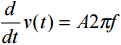The units of slew rate are volts per second and yields how fast the signal is slewing through the zero crossing of the input signal.  In a sampling system, a reference clock is used to sample the input signal.  If the sample clock has aperture uncertainty, then an error voltage is generated. This error voltage can be determined by multiplying the input slew rate by the ‘jitter’.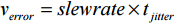By analyzing the units, it can be seen that this yields unit of volts. Usually, aperture uncertainty is expressed in seconds rms. and therefore, the error voltage would be in volts rms. Additional analysis of this equation shows that as analog input frequency increases, the rms. error voltage also increases in direct proportion to the aperture uncertainty.

In IF sampling converters clock purity is of extreme importance. As with the mixing process, the input signal is multiplied by a local oscillator or in this case, a sampling clock. Since multiplication in time is convolution in the frequency domain, the spectrum of the sample clock is convolved with the spectrum of the input signal. Since aperture uncertainty is wideband noise on the clock, it shows up as wideband noise in the sampled spectrum as well. And since an ADC is a sampling system, the spectrum is periodic and repeated around the sample rate. This wideband noise therefore degrades the noise floor performance of the ADC. The theoretical SNR for an ADC as limited by aperture uncertainty is determined by the following equation.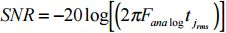If this equation is evaluated for an analog input of 201 MHz and .7 pS rms. ‘jitter’, the theoretical SNR is limited to 61 dB. It should be noted that this is the same requirement as would have been demanded had another mixer stage had been used. Therefore, systems that require very high dynamic range and very high analog input frequencies also require a very low ‘jitter’ encode source. When using standard TTL/CMOS clock oscillators modules, 0.7 pS rms. has been verified for both the ADC and oscillator. Better numbers can be achieved with low noise modules.

When considering overall system performance, a more generalized equation may be used. This equation builds on the previous equation but includes the effects of thermal noise and differential non-linearity.Although this is a simple equation, it provide much insight into the noise performance that can be expected from a data converter.

### Phase Noise

Although synthesizer phase noise is similar to jitter on the encode clock, it has slightly different effects on the receiver, but in the end, the effects are very similar. The primary difference between jitter and phase noise is that jitter is a wideband problem with uniform density around the sample clock and phase noise is a non-uniform distribution around a local oscillator that usually gets better the further away from the tone you get. As with jitter, the less phase noise the better.

Since the local oscillator is mixed with incoming signal, noise on the LO will effect the desired signal. The frequency domain process of the mixer is convolution (the time domain process of the mixer is multiplication). As a result of mixing, phase noise from the LO causes energy from adjacent (and active) channels is integrated into the desired channel as an increased noise floor. This is called reciprocal mixing. To determine the amount of noise in an unused channel when an alternate channel is occupied by a full-power signal, the following analysis is offered.

Again, since GSM is a difficult specification, this will serve as an example. In this case the following equation is valid.where Noise is the noise in the desire channel caused by phase noise, x(f) is the phase noise expressed in non-log format and p(f) is the spectral density function of the GMSK function. For this example, assume that the GSM signal power is -13 dBm. Also, assume that the LO has a phase noise that is constant across frequency (most often, the phase noise reduces with carrier offset). Under these assumptions when this equation is integrated over the channel bandwidth, a simple equation falls out. Since x(f) was assumed to be constant (PN - phase noise) and the integrated power of a full-scale GSM channel is -13 dBm, the equation simplifies to: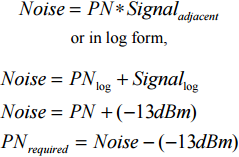Since the goal is to require that phase noise be lower than thermal noise. Assuming that noise at the mixer is the same as at the antenna, -121 dBm (noise in 200 kHz at the antenna - Pa = kTB ) can be used. Thus, the phase noise from the LO must be lower than -108 dBm with an offset of 200 kHz.

### Ссылки

Digital IF Processing,  Clay Olmstead and Mike Petrowski, TBD, September 1994, pg. 30 - 40.

Undersampling Techniques Simplify Digital Radio, Richard Groshong and Stephen Ruscak, Electronic Design, May 23, 1991, pg. 67 - 78.

Optimize ADCs For Enhanced Signal Processing, Tom Gratzek and Frank Murden, Microwaves & RF reprint.

Using Wide Dynamic Range Converters for Wide Band Radios, Brad Brannon, RF Design, May 1995, pg. 50 - 65.

Exact FM Detection of Complex Time Series, fred harris, Electrical and Computer Engineering Department, San Diego State University, San Diego, California 92182.

Introduction To Radio Frequency Design, W.H. Hayward, Prentice-Hall, 1982.

Solid State Radio Engineering, Krauss, Bostian and Raab, John Wiley & Sons, 1980.